# Physical Chemistry : Equilibrium and Kinetics

## Example Questions

← Previous 1 3 4

### Example Question #1 : Rate Law And Reaction Order

Which of the following is always true regarding reaction orders?

None of these

Rate of a reaction increases as the reaction order increases

Rate of a reaction decreases as the reaction order increases

Rate of a reaction doesn’t depend on the reaction order

Rate of a reaction doesn’t depend on the reaction order

Explanation:

Individual order of reactants is a variable used to determine the rate of a reaction. Each reactant in the rate-limiting step of a reaction is assigned an order (typically zeroth, 1st, or 2nd). The reaction order is the sum of all individual orders. The rate of a reaction is defined as follows.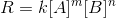Whereis the rate of the reaction,andare reactants,is rate constant, andandare individual orders. The reaction order of of this reaction would be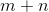. Note that the rate of a reaction depends on the concentration of reactants, rate constant, and the individual orders. It doesn’t depend on the reaction order.

### Example Question #1 : Rate Law And Reaction Order

You are analyzing a drug. After performing some tests you observe that the drug elimination follows first order. How long will it take for 75% of drug to be eliminated from the body?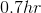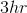Cannot be determined without knowing the rate constant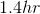Cannot be determined without knowing the rate constant

Explanation:

The half-life is defined as the amount of time it takes to reduce the concentration of a substance (in this case a drug). After one half-life, 50% of the drug will be eliminated and 50% will remain. After the second half-life, half of the remaining drug (25%) will be eliminated; therefore, at the end of second half-life 75% of drug will be eliminated. To solve for this time, we can simply calculate the half-life and multiply it by 2.

To solve this question, we need to know the equation for half-life. It is stated that the reaction follows first-order; therefore, the half-life for a first order reaction is defined as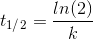Where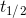is half-life andis the rate constant. Since we are not given the rate constant we cannot calculate the half-life.

### Example Question #1 : Rate Law And Reaction Order

Which of the following is true regarding a first order reaction?

The half-life increases as the concentration of reactants decreases

The half-life increases as the concentration of reactants increases

The half-life increases as the rate constant decreases

More than one of these

The half-life increases as the rate constant decreases

Explanation:

The half-life of a first order reaction is as follows:Whereis half-life andis the rate constant. The half-life only depends on the rate constant. Since rate constant is in the denominator, half-life and rate constant are inversely proportional. This means that half-life increases as rate constant decreases and vice versa. Concentration of reactants does not affect the half-life for a first order reaction.

### Example Question #1 : Rate Law And Reaction Order

The rate constant __________ as temperature increases and __________ as catalyst is added.

doesn't change . . . decreases

increases . . . increases

decreases . . . decreases

increases . . . doesn’t change

increases . . . increases

Explanation:

Rate constant is a unique constant for each reaction that determines the rate of a reaction. It is one of several variables that determines the rate of reaction. As rate constant increases the rate of reaction increases. Rate constant depends on two main factors: temperature and activation energy. The rate constant increases as the temperature is increased. Recall that increasing temperature increases the kinetic energy of the molecules; therefore, an increase in temperature will increase the amount of molecules that reach activation energy. This will increase the rate constant and, subsequently, the rate of the reaction.

Rate constant increases as the activation energy is decreased. Activation energy is the energy hill that reactants must overcome to produce products. If the activation energy is decreased then it will be easier for reactants to overcome the energy hill and convert into products; therefore, decreasing activation energy will increase the rate constant and, subsequently, the rate of the reaction. Recall that catalysts speed up a reaction (increase rate of reaction) by lowering activation energy; therefore, catalysts increase the rate constant.

### Example Question #1 : Rate Law And Reaction Order

The integrated form of the rate law for a 0th order reaction is given as: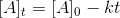Given that a reaction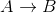with rate constantis 0th order, which of the following are false?

I. The concentration of reactant does not change with time.

II. The rate is independent of reactant concentration.

III. The reaction rate constanthas no affect on the final concentration of reactant over time.

II and III

I, II, and III

I only

I and III

II only

I and III

Explanation:

Condition I is false. The reactant concentration does change over time (can be seen the integrated rate law, which has a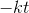term), it just does not depend on the reactant concentration.

Condition II is true. The rate is independent of reactant concentration for 0th order reactions.

Condition III is false.does have an affect on the overall reactant concentration over time (can be seen by in the integrated rate law, which has aterm).

### Example Question #1 : Rate Law And Reaction Order

The integrated rate law of a first order reaction is given by: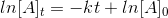where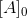is the initial reactant concentration,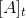is the reactant concentration at time, andis the reaction rate constant.

Which of the following are false for a first order reaction?

I. A plot of [A] vs. t will be linear

II. A plot of ln[A] vs, t will be linear

III. The rate law is proportional to ln[A]

IV. The rate law is independent of [A]

V. The rate law is proportional to [A]

II, III, and V

IV and V

II, III, and IV

I, III, and IV

I, II, III, and IV

I, III, and IV

Explanation:

The only statements that are true are that a plot of ln[A] vs t will be linear for a first order reaction (this can be seen from the integrated rate law given, since the form of the equation isWith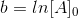and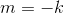and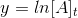Additionally, for a first order reaction, the rate is proportional to the concentration of [A].

All other statements are not true. A plot of [A] vs. t will be exponential.

### Example Question #1 : Michaelis Menton Analysis

Which of the following is true regarding the Michaelis constant?

It is the substrate concentration at which the reaction rate is half of maximum

It is the enzyme concentration at which the reaction rate is half of maximum

It is the substrate concentration at which the reaction rate is at maximum

It is the enzyme concentration at which the reaction rate is at maximum

It is the substrate concentration at which the reaction rate is half of maximum

Explanation:

Michaelis constant, or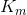, is defined as the concentration of substrate at which the reaction rate is half the maximum (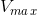). It is a useful measure of how much substrate is needed for reaction to proceed rapidly. A reaction with a high Michaelis constant will need lots of substrate to reach high reaction rates whereas a reaction with low Michaelis constant will need small amounts of substrate to reach high reaction rates.

### Example Question #1 : Equilibrium And Kinetics

Which of the following will have the greatest increase in reaction rate?

Increasing the substrate concentration by a factor of 2

Increasing the maximum velocity by a factor of 2

Increasing the Michaelis constant by a factor of 2

Decreasing the substrate concentration by a factor of 2

Increasing the maximum velocity by a factor of 2

Explanation:

Reaction rate, according to Michaelis-Menten model is as follows.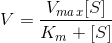whereis reaction rate,is maximum reaction rate,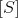is substrate concentration, andis the Michaelis constant. If we analyze the given options, we will observe that the greatest increase inoccurs whenis doubled (increased by a factor of 2). Increasing substrate concentration by a factor of 2 will have nearly the same effect; however, sinceis also found in the denominator it will only slightly contribute to an increase in.

Note that the units foris molarity,is molarity, andis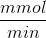. Solving forwill give us units of.

### Example Question #1 : Michaelis Menton Analysis

Consider the following reaction parameters.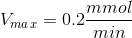Substrate concentration =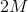Michaelis constant =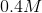What can you conclude about the reaction rate?

The reaction rate is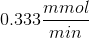The reaction rate is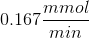The reaction rate is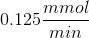The reaction rate cannot be determined from the given information

The reaction rate isExplanation:

To solve this problem we need to use the Michaelis-Menten equation.whereis reaction rate,is maximum reaction rate,is substrate concentration, andis the Michaelis constant. If we plug in the given values we get a reaction rate of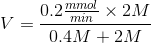Note that the Michaelis-Menten equation implies that thewill never exceed. Regardless of how high the substrate concentration is, the reaction rate will approachbut will never equal or exceed it. You can try this by substituting very high values for substrate concentration. Thewill get very close to 0.2 () but will never equal or exceed it.

### Example Question #1 : Michaelis Menton Analysis

The Michaelis-Menten model implies that __________ the Michaelis constant will __________ the reaction rate.

decreasing . . . decrease

increasing . . . decrease

increasing . . . not change

decreasing . . . not change

increasing . . . decrease

Explanation:

The Michaelis-Menten equation is as follows.Whereis reaction rate,is maximum reaction rate,is substrate concentration, andis the Michaelis constant. Since the Michaelis constant,, is in the denominator, the reaction rate is inversely proportional to the Michaelis constant; therefore, increasing the Michaelis constant will decrease the reaction rate.

← Previous 1 3 4

### All Physical Chemistry Resources